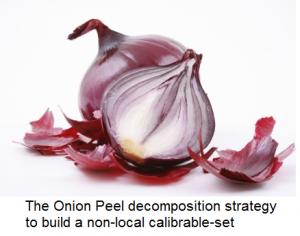# Fundamentals of Non-local Total Variation Spectral Theory

### J.-F. Aujol, G. Gilboa, N. Papadakis, “Non local total variation spectral framework”, Proc. Scale Space and Variational Methods in Computer Vision (SSVM), 2015.

Abstract:

Eigenvalue analysis based on linear operators has been extensively used in signal and image processing to solve a variety of problems such as segmentation, dimensionality reduction and more.
Recently, nonlinear spectral approaches, based on the total variation functional have been proposed. In this context, functions for which the nonlinear eigenvalue problem $\lambda u \in \partial J(u)$ admits solutions,

are studied.
When $u$ is the characteristic function of a set $A$, then it is called a calibrable set. If $\lambda >0$ is a solution of the above problem, then $1/\lambda$ can be interpreted as the scale of $A$.

However, this notion of scale remains local, and it may not be adapted for non-local features. For this we introduce in this paper the definition of non-local scale related to the non-local
total variation functional. In particular, we investigate sets that evolve with constant speed under the non-local total variation flow.
We prove that non-local calibrable sets have this property. We propose an onion peel construction to build such sets.
We eventually confirm our mathematical analysis with some simple numerical experiments.